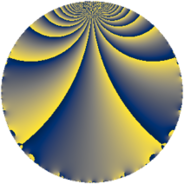# Properties

 Label 70.2.iLevel $70$ Weight $2$ Character orbit 70.i Rep. character $\chi_{70}(9,\cdot)$ Character field $\Q(\zeta_{6})$ Dimension $8$ Newform subspaces $2$ Sturm bound $24$ Trace bound $5$

# Related objects

## Defining parameters

 Level: $$N$$ $$=$$ $$70 = 2 \cdot 5 \cdot 7$$ Weight: $$k$$ $$=$$ $$2$$ Character orbit: $$[\chi]$$ $$=$$ 70.i (of order $$6$$ and degree $$2$$) Character conductor: $$\operatorname{cond}(\chi)$$ $$=$$ $$35$$ Character field: $$\Q(\zeta_{6})$$ Newform subspaces: $$2$$ Sturm bound: $$24$$ Trace bound: $$5$$ Distinguishing $$T_p$$: $$3$$

## Dimensions

The following table gives the dimensions of various subspaces of $$M_{2}(70, [\chi])$$.

Total New Old
Modular forms 32 8 24
Cusp forms 16 8 8
Eisenstein series 16 0 16

## Trace form

 $$8q + 4q^{4} + 2q^{5} - 12q^{6} + 6q^{9} + O(q^{10})$$ $$8q + 4q^{4} + 2q^{5} - 12q^{6} + 6q^{9} + 2q^{10} - 6q^{11} - 2q^{14} - 24q^{15} - 4q^{16} - 14q^{19} + 4q^{20} + 6q^{21} - 6q^{24} + 14q^{26} + 20q^{29} + 6q^{30} - 16q^{31} + 16q^{34} + 26q^{35} + 12q^{36} + 12q^{39} - 2q^{40} + 6q^{44} + 24q^{45} + 16q^{46} - 26q^{49} - 32q^{50} - 12q^{51} - 18q^{54} - 24q^{55} + 2q^{56} - 4q^{59} - 12q^{60} + 6q^{61} - 8q^{64} - 2q^{65} - 12q^{69} - 2q^{70} - 14q^{74} + 24q^{75} - 28q^{76} + 8q^{79} + 2q^{80} - 36q^{81} - 24q^{84} + 8q^{85} - 14q^{86} - 10q^{89} + 60q^{90} + 20q^{91} + 2q^{94} + 16q^{95} + 6q^{96} + 36q^{99} + O(q^{100})$$

## Decomposition of $$S_{2}^{\mathrm{new}}(70, [\chi])$$ into newform subspaces

Label Dim. $$A$$ Field CM Traces $q$-expansion
$$a_2$$ $$a_3$$ $$a_5$$ $$a_7$$
70.2.i.a $$4$$ $$0.559$$ $$\Q(\zeta_{12})$$ None $$0$$ $$0$$ $$-2$$ $$0$$ $$q+\zeta_{12}q^{2}+(-3\zeta_{12}+3\zeta_{12}^{3})q^{3}+\cdots$$
70.2.i.b $$4$$ $$0.559$$ $$\Q(\zeta_{12})$$ None $$0$$ $$0$$ $$4$$ $$0$$ $$q+\zeta_{12}q^{2}+\zeta_{12}^{2}q^{4}+(2-\zeta_{12}-2\zeta_{12}^{2}+\cdots)q^{5}+\cdots$$

## Decomposition of $$S_{2}^{\mathrm{old}}(70, [\chi])$$ into lower level spaces

$$S_{2}^{\mathrm{old}}(70, [\chi]) \cong$$ $$S_{2}^{\mathrm{new}}(35, [\chi])$$$$^{\oplus 2}$$